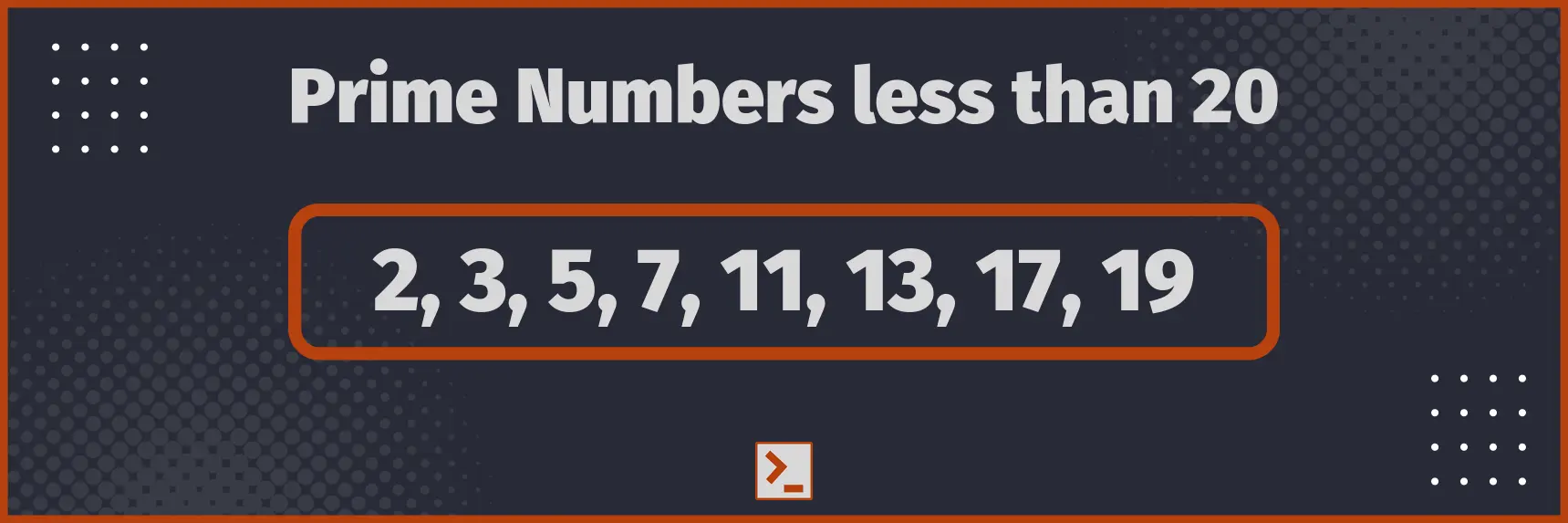# Prime Numbers

and program to check if a number is Prime number or not in Rust.

## What are the Prime Numbers ?

Prime Numbers are the natural number greater than 1, that are divisible only by 1 and the number itself.

Alternatively, you can say that prime numbers are the natural numbers greater than 1 that have only 2 factors, that is 1 and the number itself.

Smallest Prime number is 2.

For Example : 19 is a prime number. It is only divisible by 1 and 19 itself.## Properties of Prime Numbers

• All prime numbers except 2 are odd numbers. 2 is also the smallest prime number.
• 2 prime numbers are always co prime to each other, that is their GCD = 1.
• Every natural number greater than 1 can be expressed as product of powers of prime numbers, which is unique. See Prime Factorization of a number
• Every even natural number greater than 2 can be expressed as the sum of two prime numbers.
• Fermat's little theorem, for any number n, and a prime number p, ( n p-1 ) mod p = 1
• Every prime number greater than 3 can be written as 6n+1 or 6n-1, where n is any natural number.

## Check if a number is Prime

Let us now make a function to check if a given number is prime or not. We iterate through all the number from 2 to square root of number and check if it divides the number. If yes, return false.

Finally, return true because it not divisible by any number.

Proof

Let us suppose, that some number, N is not prime. So, we can write it as

N = A × B

and 1 < A ≤ B < N. So, A must be less than or equal to square root of N, else A × B will become greater than N, because A × A > N and A ≤ B => A × B > N.

Hence, we have to check for the numbers only upto sqrt( N ).

### Program

```fn check_prime(n:usize) -> bool{
// Iterate from i = 2 to sqrt ( n )    let mut i:usize = 2;    while i*i<=n {        // Return false if the number is divisible        if n%i == 0 {            return false;        }        i+=1;    }
// Return true finally    return true;}```

with Driver code

```use std::io::stdin;
fn take_int() -> usize {    let mut input = String::new();    stdin().read_line(&mut input).unwrap();    return input.trim().parse().unwrap();}
fn check_prime(n:usize) -> bool{
// Iterate from i = 2 to sqrt ( n )    let mut i = 2;    while i*i<=n {        // Return false if the number is divisible        if n%i == 0 {            return false;        }        i+=1;    }
// Return true finally    return true;}
pub fn main() {    // Take the number from user    let n = take_int();
// Output on the basis of if the number is prime or not
if check_prime(n) {        println!("Yes, given number is a prime number");    }    else {        println!("No, given number is not a prime number");    }}```

Input

1000000007

Output

Yes, given number is a prime number

Time Complexity : O( sqrt( N ) )
Space Complexity : O( 1 )

## Conclusion

Prime numbers is a very important concept, especially from Competitive programming point of view. They have plenty of interesting properties and interesting patterns.

In this article, we discussed some of the properties of prime numbers, as well as made a program to check whether a given number is prime or not in square root time complexity.

Here is the optimized function for easy access

`fn check_prime(n:usize) -> bool{    let mut i = 2;    while i*i<=n {        if n%i == 0 { return false; }        i+=1;    }    return true;}`

Thank You